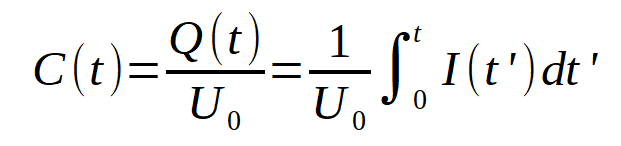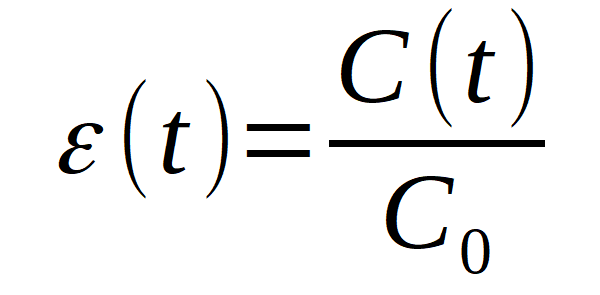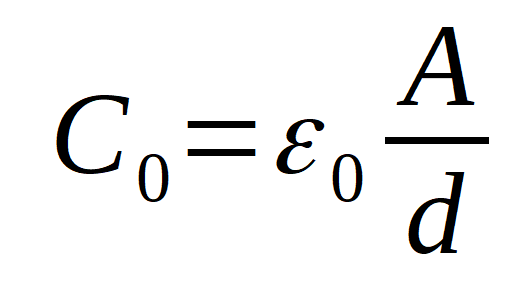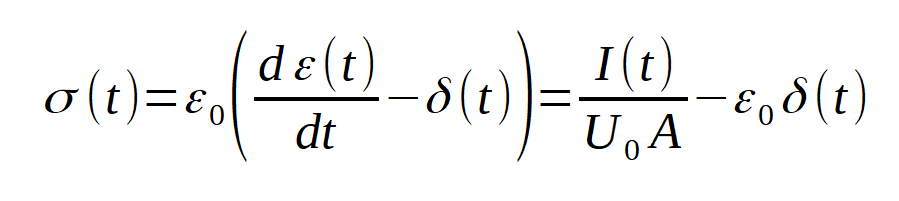Excellence in Broadband Dielectric Spectroscopy • Impedance Spectroscopy • Conductivity Spectroscopy

# Time Domain Measurement

The purpose is to measure the permittivity ε(t) and conductivity σ(t) functions  of a sample material in dependence of time t. The material may be solid or liquid and is usually placed between two electrodes which form a capacitor.Fig. 1: Principle of a time domain dielectric and conductivity measurement.

At time t=0, voltage step from 0 V to U0 is applied to the sample capacitor. The response current I(t) is measured as a function of time. From this, the time dependent capacity is calculated fromIt is directly related to the time dependent permittivity function bywhere C0 is the capacity of the empty electrodes without sample material in between. If the electrodes are arranged in parallelwhere A denotes the area of one electrode, d the spacing between the electrodes and ε0=8.854*10-12 As/Vm is the vacuum permittivity.;

It should be noted, that ε(t) generally contains a permittivity contribution from molecular dipoles and a conductivity contribution from free charge carriers. The same applies to the time dependent conductivity function, which is another representation of ε(t)The delta function δ(t) takes the current peak of the empty electrodes (vacuum permittivity) at t=0 into acount, resulting in ε(t) = 1 and σ(t) = 0 for the vacuum. Refer to Dielectric Spectroscopy and Impedance Spectroscopy of Materials for details and typical properties of ε(t) and σ(t).